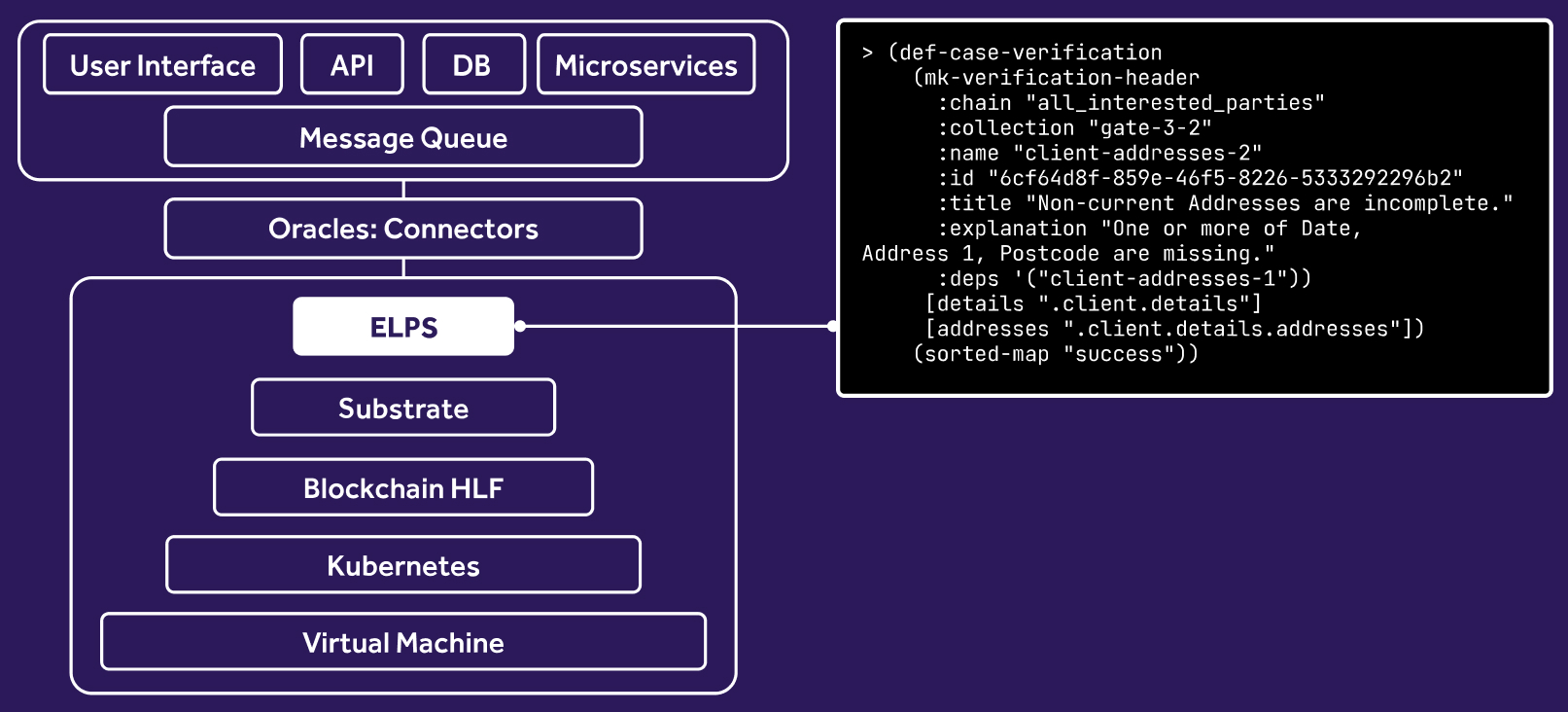# 6.2.3. Standard Library

Reference for builtin functions.

## Function Reference

``````debug-print
``````

Prints the supplied value(s).

`	                                            `
`	                                            `
``````json:dump-string
``````

JSON encodes a value, returning the JSON as a string.

`	                                            `
`	                                            `
``````json:dump-bytes
``````

JSON encodes a value, returning the JSON as bytes.

`	                                            `
`	                                            `
``````to-string
``````

Converts primitive values to their string representation.

`	                                            `
`	                                            `
``````to-bytes
``````

Converts a string value to bytes.

`	                                            `
`	                                            `
``````to-int
``````

Converts string digits ([0-9]+) and floats to integers. For floats the fractional part is discarded. Allows integer to pass through.

`	                                            `
`	                                            `
``````to-float
``````

Converts strings and integers to floats. Allows floats to pass through.

`	                                            `
`	                                            `
``````car
``````

Returns the first element in a list.

`	                                            `
`	                                            `
``````cdr
``````

Returns the list after the first item.

`	                                            `
`	                                            `
``````rest
``````

Returns the sequence (list, vector) after the first item.

`	                                            `
`	                                            `
``````first / second
``````
`	                                            `
`	                                            `
``````nth
``````

Gets the nth element of a sequence.

`	                                            `
`	                                            `
``````map
``````

Applies a function to a sequence of values.

`	                                            `
`	                                            `
``````foldl
``````

Reduces a sequence using an applicator function and with an accumulator, evaluating from the left.

`	                                            `
`	                                            `
``````foldr
``````

Reduces a sequence using an applicator function and with an accumulator, evaluating from the right.

`	                                            `
`	                                            `
``````compose
``````

Returns a function that is the combination of two functions, with the first function using the product of the second function as its input.

`	                                            `
`	                                            `
``````unpack
``````

Unpack is a special case of apply and is more cumbersome. Instead of using unpack it is better to use apply and funcall.

`	                                            `
`	                                            `
``````flip
``````

Returns a function with the parameter order reversed, the input function must have two parameters.

`	                                            `
`	                                            `
``````assoc
``````

Associates a new key and value to a map, returning a copy without mutating the source map.

`	                                            `
`	                                            `
``````assoc!
``````

Associates a new key and value to a map, mutating the source map in-place.

`	                                            `
`	                                            `
``````dissoc
``````

Dissociate a value from a map via a key, returning a copy without mutating the source map.

`	                                            `
`	                                            `
``````dissoc!
``````

Dissociate a value from a map via a key, mutating the source map in-place.

`	                                            `
`	                                            `
``````get
``````

Gets a map value by key.

`	                                            `
`	                                            `
``````keys
``````

Returns the key values of a map.

`	                                            `
`	                                            `
``````key?
``````

Checks if the a key exists in a map.

`	                                            `
`	                                            `
``````concat
``````

Concatenates values.

`	                                            `
`	                                            `
``````insert-index
``````

Inserts a value into a sequence at a specific index.

`	                                            `
`	                                            `
``````stable-sort
``````

Performs a stable sort on a list using a predicate. The last argument can optionally be a function that takes the key and returns the comparison value. Mutates the list in-place.

`	                                            `
`	                                            `
``````insert-sorted
``````

Inserts a value in its sort position.

`	                                            `
`	                                            `
``````search-sorted
``````

Search uses binary search to find and return the smallest index i in [0, n) at which f(i) is true, assuming that on the range [0, n), f(i) == true implies f(i+1) == true.

`	                                            `
`	                                            `
``````select
``````

Selects values matching the predicate.

`	                                            `
`	                                            `
``````reject
``````

Rejects values matching the predicate.

`	                                            `
`	                                            `
``````zip
``````

Zips one or more lists, composing a list of values from each input list. Tuples length is restricted to the smallest input list length.

`	                                            `
`	                                            `
``````make-sequence
``````

Generates a sequence, with an optional step value.

`	                                            `
`	                                            `
``````format-string
``````

Creates a string using format placeholders and values.

`	                                            `
`	                                            `
``````reverse
``````

Reverses a sequence.

`	                                            `
`	                                            `
``````slice
``````

Returns the sub-slice of a sequence.

`	                                            `
`	                                            `
``````list
``````

Returns a list compose of the supplied parameters.

`	                                            `
`	                                            `
``````vector
``````

Creates a vector (array) value.

`	                                            `
`	                                            `
``````append
``````

Appends to the vector, returning a copy without mutating the source vector.

`	                                            `
`	                                            `
``````append!
``````

Appends to the vector, mutating the source vector in-place.

`	                                            `
`	                                            `
``````append-bytes
``````

Appends to the byte vector, returning a copy without mutating the source vector.

`	                                            `
`	                                            `
``````append-bytes!
``````

Appends to the byte vector, mutating the source vector in-place.

`	                                            `
`	                                            `
``````aref
``````

Gets the array element at the given index (counted from zero).

`	                                            `
`	                                            `
``````all?
``````

Test all items in a sequence match against a function.

`	                                            `
`	                                            `
``````any?
``````

Test if any item in a sequence matches against a function.

`	                                            `
`	                                            `
``````true?
``````

Checks if a value is truthy.

`	                                            `
`	                                            `

## Type Checking

`	                                            `
`	                                            `

### empty?

Determine if a sequence or string is empty.

`	                                            `
`	                                            `